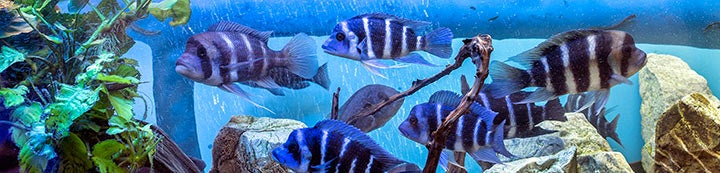1 - 2 of 2 Posts

Gravel Cleaner

·
Registered
Joined
·
1 Posts
Discussion Starter · ·
Hi Fellow fish collectors!

Happy New year!

I have a question, I just finished setting up my 2nd tank which is a 33gal and what I read mostly says that they need a min of 50 gallon tank to keep Aulonocara Firefish - Dragonfish. I was wondering if anyone has kept these fish in a 33 and what you all think. I would keep just those in the tank.

Thanks !

GC

<º))))><`· <º))))><`· <º))))><`· <º))))><`· <º))))><
<º))))><`· <º))))><`· <º))))><`· <º))))><`· <º))))><
<º))))><`· <º))))><`· <º))))><`· <º))))><`· <º))))><
<º))))><`· <º))))><`· <º))))><`· <º))))><`· <º))))><

DJRansome

·
Super Moderator
Joined
·
40,883 Posts
I would do 48x18 (which is usually a 75G) for a hybrid peacock like a firefish/dragonfish. What are the dimensions of your 33G? Some 30G are 30" and some are 48" long but I have never heard of a 30G that is 48x18.

You already have them in the 33G so why not see what happens and report back? Maybe you got some shy ones. :thumb:

1 - 2 of 2 Posts